# Excel 2010: Radians and Degrees Function

When dealing with spreadsheet which mainly includes data in radians and degrees, it would be great to make use of Excel inherent function to play around with them. Excel RADIANS & DEGREES functions allows you to convert values either in Radians or Degrees. With these simple functions you can now change values in desired form, rather than being caught-up in applying complex formulas manually.

Launch Excel 2010 spreadsheet on which you want to change values in to Degrees. For instance we have included spreadsheet containing Radians field, as shown in the screenshot below.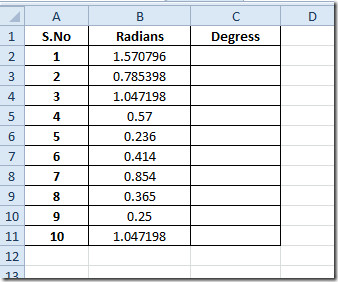Now we want to convert radians into degree value, as you might have been acquaint with the generic formula that is used for converting values in to degrees;

Where Pi is a constant (3.14159), for using this formula in Excel we don’t need to enter it manually. Excel has an inherent function for this conversion.

The syntax of the function is;

=DEGREES(angle)

Where the single argument required is angle, so we will be writing this formula in the first row of Degrees field;

=DEGREES(B2)

B2 is the location of the cell that contain values in radians.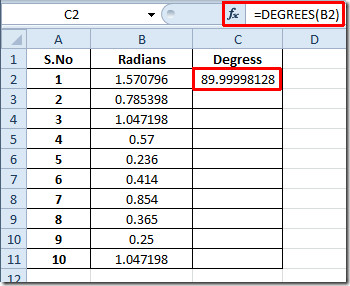Now we need to apply it over the Degrees column to find out all the corresponding values in degrees. For this, drag the plus sign at the end of the cell towards the end of the column.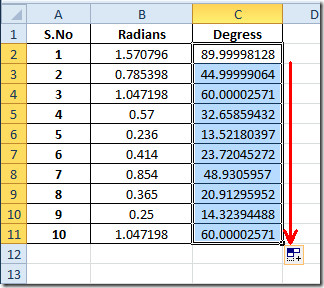If you want to know the corresponding degree value for a radian, then you can write it exclusively as an argument.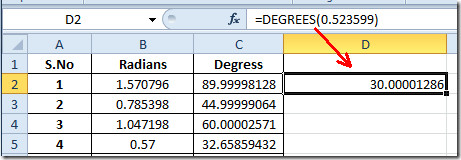If you are dealing with the datasheet that contains degree value and you want to convert them in to radians, then Excel Radian function would help you in the same way as it did for degrees values.

You need to write it as RADIANS(degree_value). As you can see in the screenshot below, that we have specified location of the cell as argument.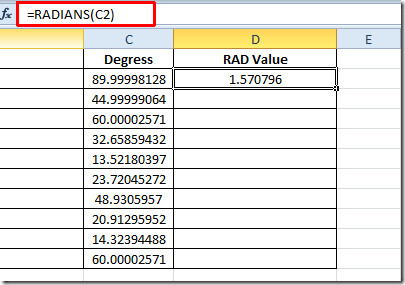Apply it over to see all the respective values in radians.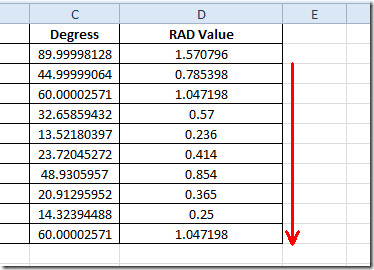You can also check out previously reviewed Excel Functions; SUMPRODUCT, SUMIF, COUNTIF, VLOOKUP, HLOOKUP ,PMT, and LEN.

1.Thomas Hall says: